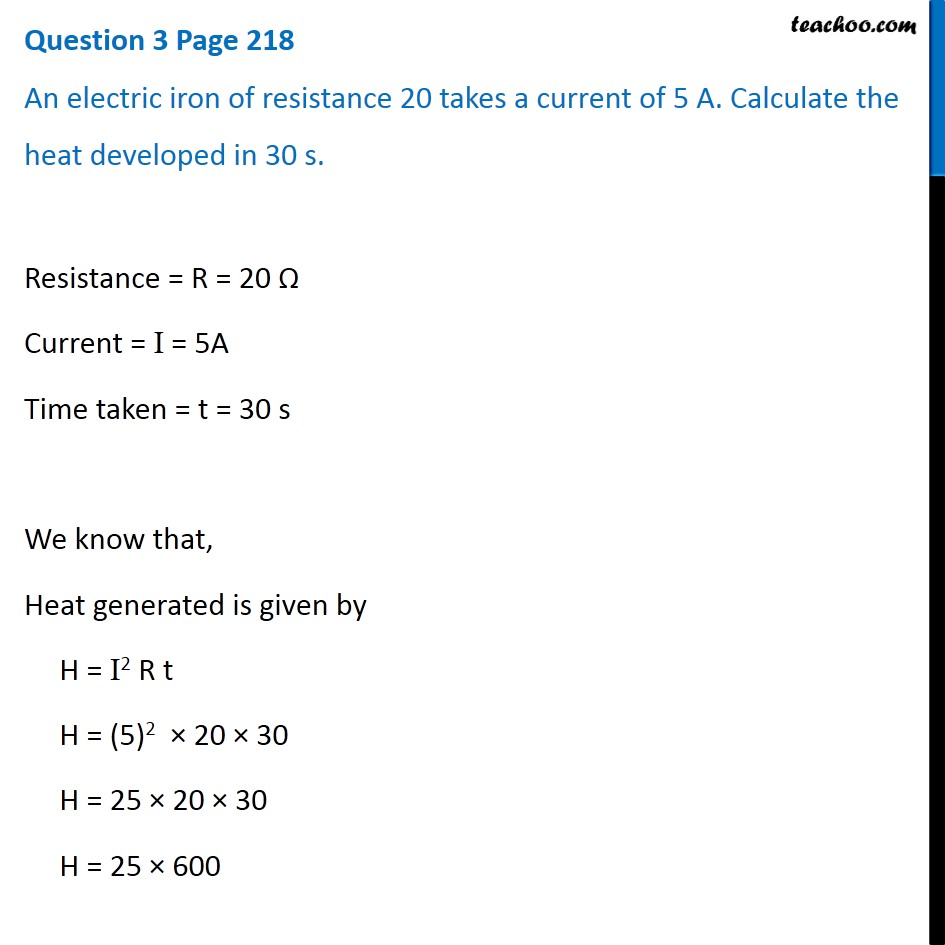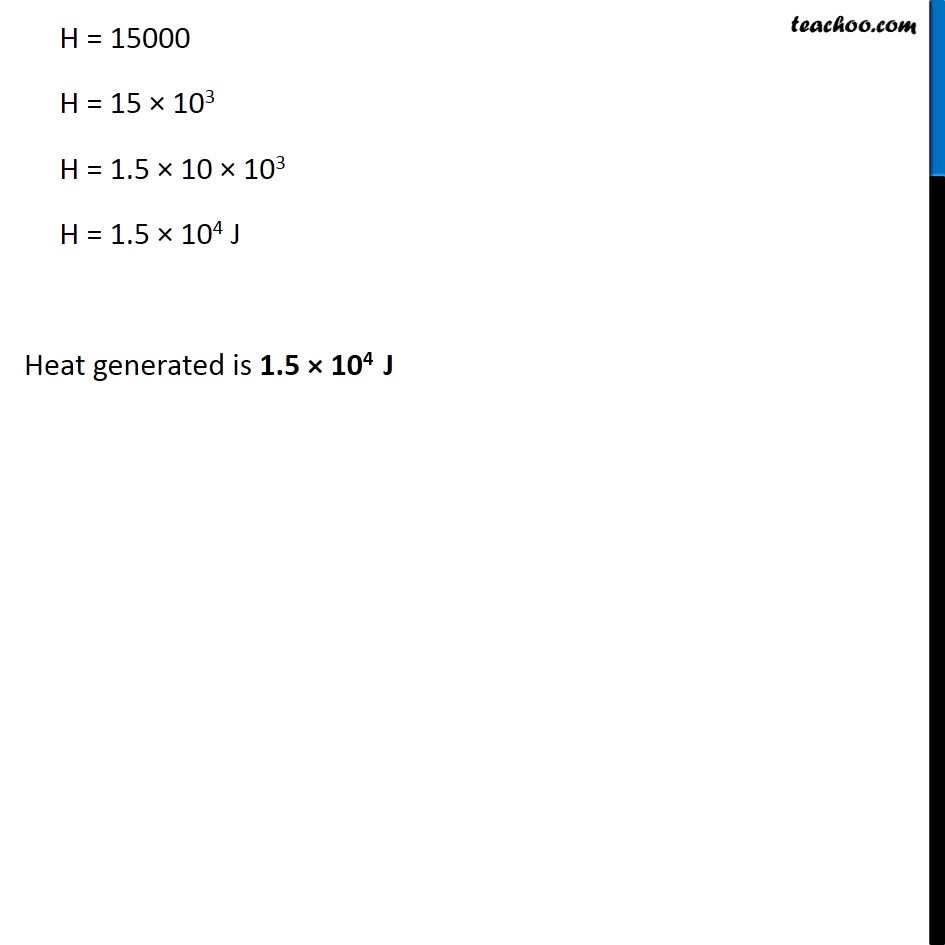Questions from Inside the chapter

Class 10
Chapter 12 Class 10 - Electricity

## An electric iron of resistance 20 Ω takes a current of 5 A. Calculate the heat developed in 30 s.Get live Maths 1-on-1 Classs - Class 6 to 12

### Transcript

Question 3 Page 218 An electric iron of resistance 20 takes a current of 5 A. Calculate the heat developed in 30 s. Resistance = R = 20 Ω Current = I = 5A Time taken = t = 30 s We know that, Heat generated is given by H = I2 R t H = (5)2 × 20 × 30 H = 25 × 20 × 30 H = 25 × 600 H = 15000 H = 15 × 103 H = 1.5 × 10 × 103 H = 1.5 × 104 J Heat generated is 1.5 × 104 J